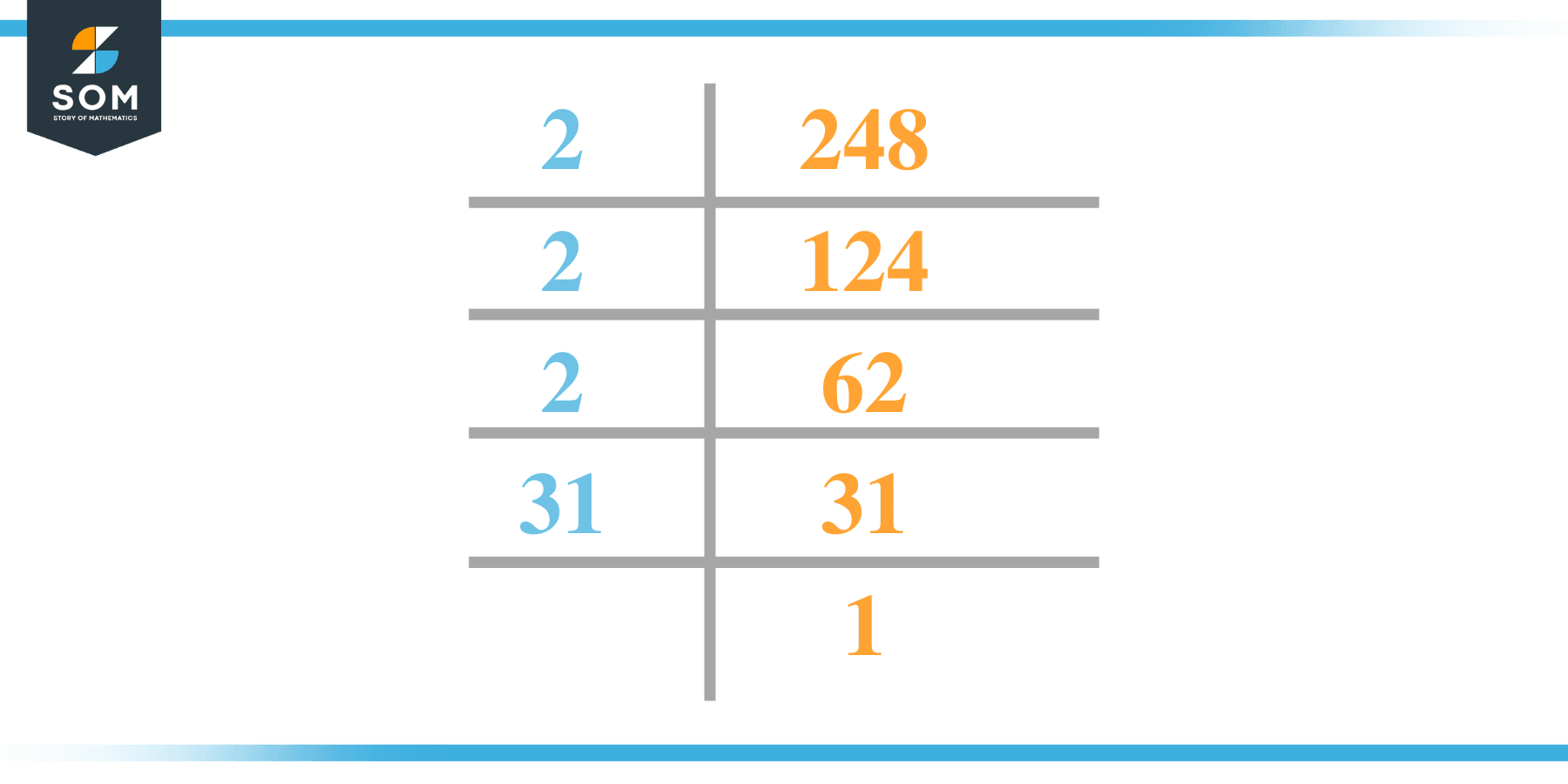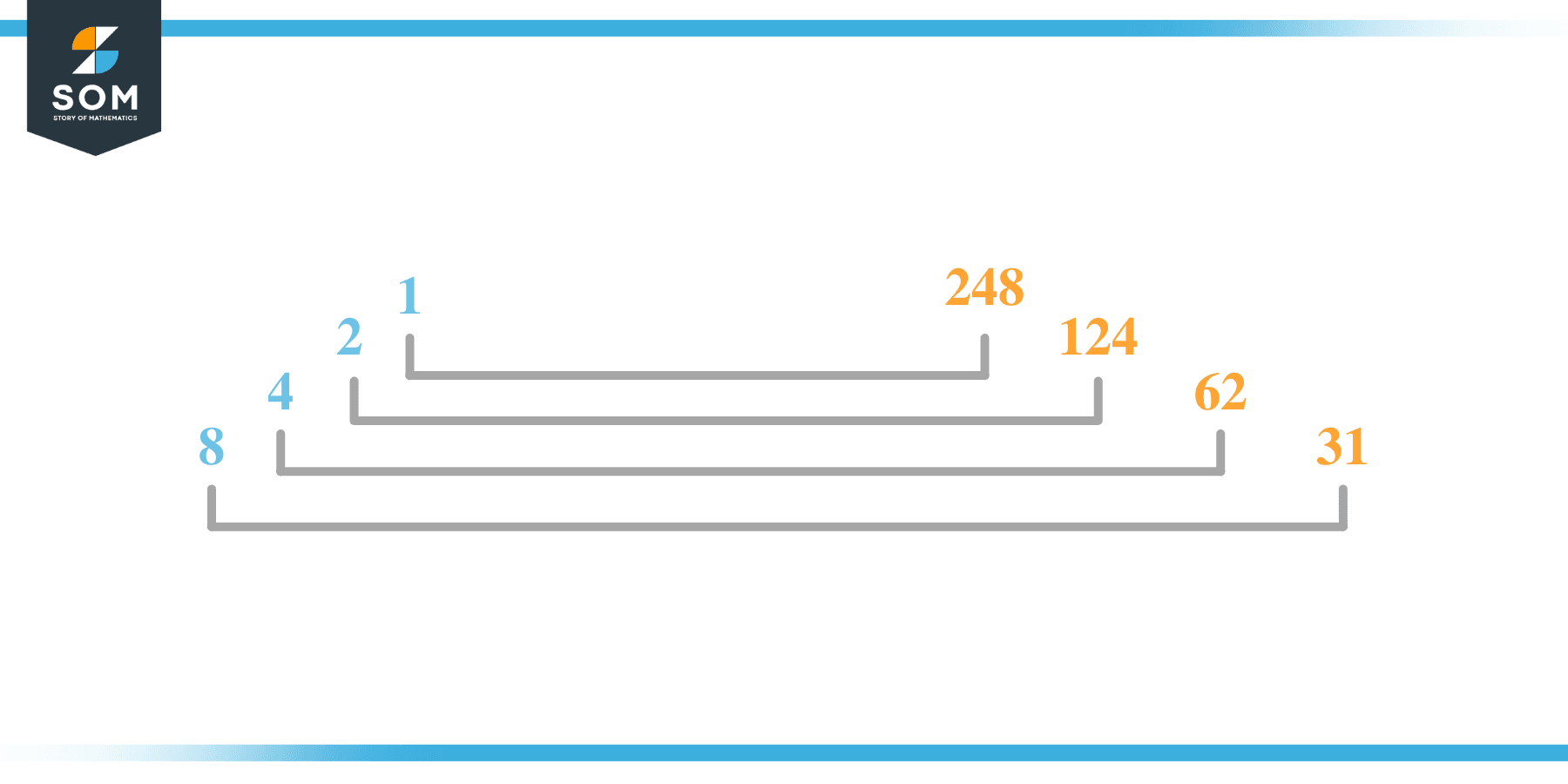# Factors of 248: Prime Factorization, Methods, and Examples

The factors of 248 are numbers that give 248 as the product when multiplied together.The given number’s factors can be positive and negative, provided that the given number is achieved upon multiplication of two-factor integers.

### Factors of 248

Here are the factors of number 248.

Factors of 248: 1, 2, 4, 8, 31, 62, 124 and 248

### Negative Factors of 248

The negative factors of 248 are similar to its positive factors, just with a negative sign.

Negative Factors of 248: -1, -2, -4, -8, -31, -62, -124 and -248

### Prime Factorization of 248

The prime factorization of 248 is the way of expressing its prime factors in the product form.

Prime Factorization = 2$^{3}$ x 31

In this article, we will learn about the factors of 248 and how to find them using various techniques such as upside-down division, prime factorization, and factor tree.

## What Are the Factors of 248?

The factors of 248 are 1, 2, 4, 8, 31, 62, 124, and 248. All of these numbers are the factors as they do not leave any remainder when divided by 248.

The factors of 248 are classified as prime numbers and composite numbers. The prime factors of the number 248 can be determined using the prime factorization technique.

## How To Find the Factors of 248?

You can find the factors of 248 by using the rules of divisibility. The divisibility rule states that any number, when divided by any other natural number, is said to be divisible by the number if the quotient is the whole number and the resulting remainder is zero.

To find the factors of 248, create a list containing the numbers divisible by 248 with zero remainders. One important thing to note is that 1 and 248 are the 248’s factors as every natural number has one and the number itself as its factor.

One is also called the universal factor of every number. The factors of 248 are determined as follows:

$\dfrac{248}{1} = 248$

$\dfrac{248}{2} = 124$

$\dfrac{248}{4} = 62$

$\dfrac{248}{8} = 31$

Therefore, 1, 2, 4, 8, 31, 62, 124, and 248 are the factors of 248, along with their whole number quotients.

### Total Number of Factors of 248

For 248, there are eight positive factors and eight negative ones. So in total, there are 16 factors of 248.

To find the total number of factors of the given number, follow the procedure mentioned below:

1. Find the factorization of the given number.
2. Demonstrate the prime factorization of the number in the exponent form.
3. Add 1 to each of the exponents of the prime factor.
4. Now, multiply the resulting exponents together. This obtained product is equivalent to the total number of factors of the given number.

By following this procedure, the total number of factors of 248 is given as:

Factorization= 1 x 2$^{3}$ x 31

The exponent of 1 and 31 is one, and the exponent of 2 is 3

Adding 1 to each and multiplying them together results in 16.

Therefore, the total number of factors of 248 is 16. 8 are positive, and eight are negative.

### Important Notes

Here are some essential points that must be considered while finding the factors of any given number:

• The factor of any given number must be a whole number.
• The number factors cannot be in the form of decimals or fractions.
• Factors can be positive as well as negative.
• Negative factors are the additive inverse of the positive factors of a given number.
• The factor of a number cannot be greater than that number.
• Every even number has two as its prime factor, the smallest prime factor.

## Factors of 248 by Prime FactorizationThe number 248 is a composite number. Prime factorization is a valuable technique for finding the number’s prime factors and expressing the number as the product of its prime factors.

Before finding the factors of 248 using prime factorization, let us find out what prime factors are. Prime factors are the factors of any given number that are only divisible by 1 and themselves.

To start the prime factorization of 248, divide by its smallest prime factor. First, determine that the given number is either even or odd. If it is an even number, then 2 will be the smallest prime factor.

Continue splitting the quotient obtained until 1 is received as the quotient. The prime factorization of 248 can be expressed as:

248 = 2$^{3}$ x 31

## Factors of 248 in PairsThe factor pairs are the duplet of numbers that when multiplied together, result in the factorized number. Factor pairs can be more than one depending on the total number of factors.

For 248, the factor pairs can be found as:

1 x 248 = 248

2 x 124 = 248

4 x 62 = 248

8 x 31 = 248

The possible factor pairs of 248 are given as (1, 248), (2, 124), (3, 62), and (4, 31).

All these numbers in pairs, when multiplied, give 248 as the product.

The negative factor pairs of 248 are given as:

-1 x -248 = 248

-2 x -124 = 248

-4 x -62 = 248

-8 x -31 = 248

It is important to note that in negative factor pairs, the minus sign has been multiplied by the minus sign, due to which the resulting product is the original positive number. Therefore, -1, -2, -4, -31, -62, -124, and -248 are called negative factors of 248.

The list of all the factors of 248, including positive and negative numbers is given below.

Factor list of 248: 1, -1, 2, -2, 4, -4, 8, -8, 31, -31, 62, -62, 124, -124, 248 and -248

## Factors of 248 Solved Examples

To better understand the concept of factors, let’s solve some examples.

### Example 1

How many factors of 248 are there?

### Solution

The total number of Factors of 248 is 16.

Factors of 248 are 1, 2, 4, 8, 31, 62, 124 and 248.

### Example 2

Find the factors of 248 using prime factorization.

### Solution

The prime factorization of 248 is given as:

248 $\div$ 2 = 124

124 $\div$ 2 = 62

62 $\div$ 2 = 31

31 $\div$ 31 = 1

So the prime factorization of 248 can be written as:

2$^{3}$ x 31 = 248